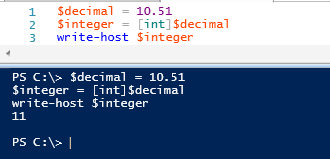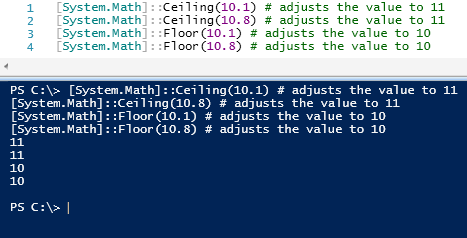# Convert Decimal number to Integer using Powershell

While writing Powershell scripts we come across the need for converting numbers from one number system to another. In this post, I will show you how to convert a decimal number to Integer.

The easiest way to do this in Powershell is, using the [int] accelerator. Look at the below example for more clarity.

```\$decimal = 10.51
\$integer = [int]\$decimal
write-host \$integer```Another way available is to use the System.Math dotnet class which has functions to adjust a decimal to nearest integer. For example, if your decimal value is 10.12, you can either adjust it to 10 or to 11. Based on your requirement, you can use one of Ceiling() or Floor() methods of Math class. See below example for more details.

```[System.Math]::Ceiling(10.1) # adjusts the value to 11
[System.Math]::Ceiling(10.8) # adjusts the value to 11
[System.Math]::Floor(10.1) # adjusts the value to 10
[System.Math]::Floor(10.8) # adjusts the value to 10```

## Output:Hope these examples helps you in doing the conversions. Happy learning.